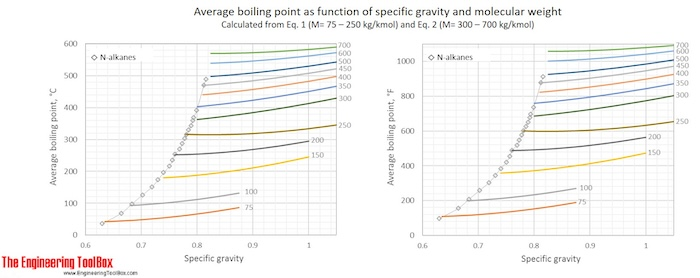Engineering ToolBox - Resources, Tools and Basic Information for Engineering and Design of Technical Applications!

# Hydrocarbon Mixtures - Average Boiling Points vs. Gravity and Molecular Weights

## Formulas and examples of calculation of boiling point of hydrocarbon mixtures from gravity and molecular weight.

Boiling point: The temperature at which a liquid turns into a gas

For many purposes in chemical engineering, there is important to know the boiling point of the fluids in process streams. When the fluid consists of a mixture of many different compounds, the average boiling point (ABP) can be useful. But boiling point information from real distillation or gas chromatographic analysis is not always available. However, there are several models calculating average boiling point, based on other properties of the fluid.  For hydrocarbons, such as crude oil and distillation fractions of crude oil, the best input pair of parameters to predict boiling point is molecular weight and specific gravity. Two correlations based on these from Riazi-Daubert are given below:

Riazi-Daubert, for  molecular weights of 70-300:    Tb = 3.76587*e(3.7741E-03*M+2.98404*S-4.25288E-03*M*S)*M0.40167*S-1.58262         (1)

Riazi-Daubert, for molecular weights of 300-700:   Tb = 9.3369*e(1.6514E-04*M+1.4103*S-7.5152E-04*M*S)*M0.5369*S-0.7276                  (2)
Eq. (2) is also applicable to hydrocarbons having molecular weight range of 70-300, but with less accuracy

Where
Tb = Average boiling point, in Kelvin
M = Average molecular weight
S = Specific gravity at 60 °F  (= 15.6°C)

Eq. (1) and (2) are used to calculate Tb for a number of specific gravities and molecular weights and the results, in °C and °F, are given in the figures below.Example 1:  Average boiling point of naphtha
Calculate the average boiling point of a naphtha with specific gravtity,  S = 0.763  and a molecular weight of 125.

Naphtha is in the low molecular weight range, and equation (1) should be applied.

ABP = 3.76587*e(3.7741E-03*125+2.98404*0.763-4.25288E-03*125*0.763)*1250.40167*0.763-1.58262 = 418 K

Converted to °C and °F: 418 K = 145°C = 293 °F

Applying equation (2) gives

ABP =  9.3369*e(1.6514E-04*125+1.4103*0.763-7.5152E-04*125*0.763)*1250.5369*0.763-0.7276 = 423 K = 150°C = 302°F

NB! For low molecular weights (< 300) this ABP is consider to be less accurate than calculated from Eq. (1).

Example 2: Average boiling point of a vacuum gas oil.
Calculate the average boiling point of a vacuum gas oil of gravity of 16.7°API and an average molecular weight of 391.

The vacuum gas oil has a molecular weight > 300, and Eq. (2) must be applied.

But first, we have to convert the API gravity to specific gravity:

S, specific gravity = 141.5/(16.7°API +131.5) = 0.955

ABP =  9.3369*e(1.6514E-04*391+1.4103*0.955-7.5152E-04*391*0.955)*3910.5369*0.955-0.7276 = 737 K = 464°C = 867°F

## Related Topics

• Fluid Mechanics - The study of fluids - liquids and gases. Involving velocity, pressure, density and temperature as functions of space and time.
• Material Properties - Material properties of gases, fluids and solids - densities, specific heats, viscosities and more.
• Boiling Points - Boiling points of elements, products and chemical species at varying conditions.

## Engineering ToolBox - SketchUp Extension - Online 3D modeling!

Add standard and customized parametric components - like flange beams, lumbers, piping, stairs and more - to your Sketchup model with the Engineering ToolBox - SketchUp Extension - enabled for use with the amazing, fun and free SketchUp Make and SketchUp Pro .Add the Engineering ToolBox extension to your SketchUp from the SketchUp Pro Sketchup Extension Warehouse!

Translate

## Privacy

We don't collect information from our users. Only emails and answers are saved in our archive. Cookies are only used in the browser to improve user experience.

Some of our calculators and applications let you save application data to your local computer. These applications will - due to browser restrictions - send data between your browser and our server. We don't save this data.

## Citation

• Engineering ToolBox, (2017). Hydrocarbon Mixtures - Average Boiling Points vs. Gravity and Molecular Weights. [online] Available at: https://www.engineeringtoolbox.com/average-boiling-point-mixtures-calculation-prediction-estimation-gravity-density-molecular-weight-d_1996.html [Accessed Day Mo. Year].

Modify access date.

. .

#### Scientific Online Calculator6 24